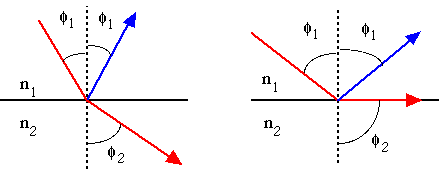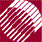ECE532 Biomedical Optics © 1998 Steven L. Jacques, Scott A. Prahl Oregon Graduate Institute

# Collection by an optical fiber

An optical fiber can guide rays of light due to multiple internal reflectance at the interface between the central fiber core (refractive index n1) and the surrounding cladding (refractive index n2). (More about REFRACTIVE INDEX in the next class.)

Consider an optical fiber collecting light from its surroundings, as shown in the following figure. The fiber is a central core of glass surrounding by a polymer cladding and is collecting light from the LEFT in the air.

A ray of light enters the glass core of the fiber at its open end (on the left) at an angle θ1 relative to the fiber axis. The angle of incidence is bent by refraction at the air/glass interface into a new angle θ2 which is oriented more closely to the fiber axis. (More about REFRACTION next class.) The ray obliquely (angle φ1) strikes the glass/polymer interface of the core/cladding surface and is internally reflected . (More about INTERNAL REFLECTANCE next class.) The ray continues to be multiply internally reflected as it propagates down the fiber to the RIGHT. Rays that enter the fiber from outside the cone of collection will not be successfully internally reflected and will escape the fiber and not propagate.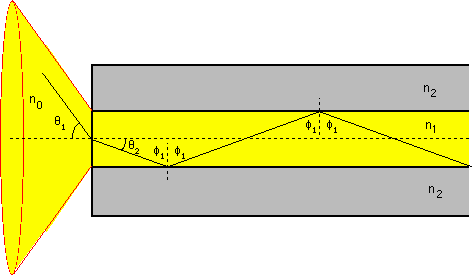The situation with the optical fiber is equivalent to the aperture with restricted solid angle of collection which was discussed earlier. Only power flowing into the fiber within the acceptance angle of the fiber will be internally reflected within the fiber and successfully transmitted down the fiber to some detection system. In general, the acceptance angle θa depends on the values of n0, n1 and n2 according to the expression: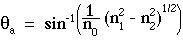EXAMPLE: Assume typical fiber conditions:

• the medium surrounding the fiber is air with a refractive index n0 = 1.0
• the core is glass, n1 = 1.50
• the cladding is a polymer, n2 = 1.47.
• the acceptance angle is:
θa = sin-1( (1/1.0)(1.502 - 1.472)1/2 ) = 17.4°
• The solid angle of collection is:
Ω = π(r/R)2   (see previous figure and equation)

= π(tan(θa))2 = 0.984 [sr]

### Brief introduction to Snell's Law

(More about SNELL's LAW next class.)

Snell's Law describes the angle of transmission (φ2) of a light ray in response to an angle of incidence (φ1) at an interface between two media with different refractive indices. The angle of reflectance is equal in magnitude to the incident angle.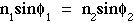• The LEFT figure below shows the basic rule. A ray of light is incident at angle φ1 relative to the normal to the interface. The ray transmitted into medium 2 from medium 1 is turned into a new angle φ2. In this example, n2 < n1.
• The RIGHT figure shows the special case of incidence at the critical angle called φc at which the transmitted ray is aligned with the interface. There is zero transmission into medium 2. At any angle φ1 > φc, there is no transmission and the ray is totally internally reflected into medium 1 at an angle of magnitude φ1 opposite the incident angle.